# An Introduction to a Most Sagacious Sumo

Author: Cameron Ditchfield

For a logging and monitoring system to provide the most amount of value, its front end must be easily accessible and understandable to the developers and administrators who may only have cause to interact with it once in a blue moon. We have recently adopted Sumo Logic alongside our existing tools with the intention of replacing them. Sumo Logic is a web service that serves as a central collection point for all your logs and metrics, and aims to provide a friendly but easy-to-use interface into the formidable quantities of data generated by your systems. With Sumo Logic, you can monitor your logs and metrics using scheduled searches to alert your teams to important events. This post will provide a short, high-level introduction to some of Sumo Logic’s features, with a few thoughts on how it performs and what we hope Sumo Logic can do for us in the future.

Because Sumo Logic is meant to graphically display your logs and metrics, most interactions are done through the web interface. It is from here that queries are written, dashboards viewed and data ingestion configured. Sumo Logic ingests data using “collectors” which come in two types: installed and hosted. Installed collectors are java programs that are installed on your servers to collect logs and metrics from local sources, although they can also do things like run a syslog server or access files over SSH. Hosted collectors are a mixed bag; they can provide you with a URL so that you can send data directly from your sources, or they can integrate directly with other services to collect data from Google Cloud or Amazon Web Services.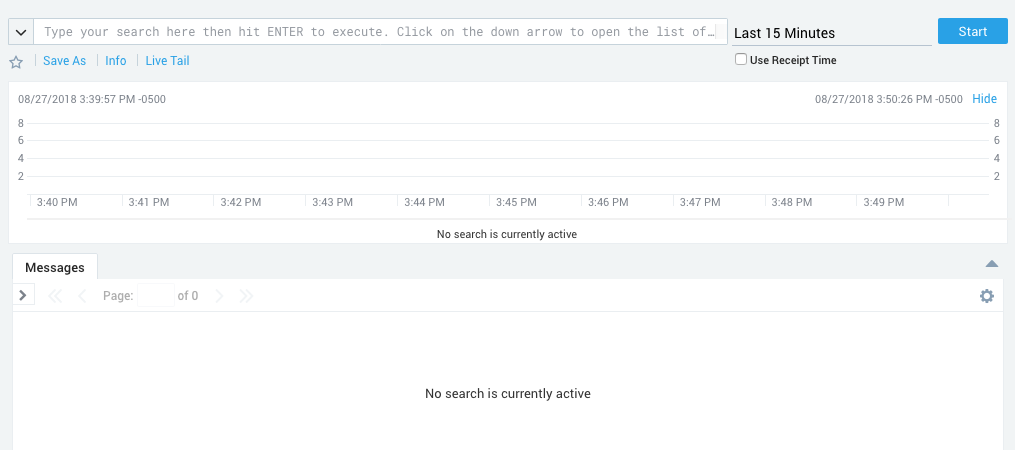So that you may view your logs, Sumo Logic provides an interface containing a bar graph that breaks down the times when you received the most number of messages, a text field to enter a search, and a stream displaying all the matching logs. The most basic searches match a string against the body of the logs, but further refinement is easy. Sumo Logic keeps some metadata about your log sources by default, and keywords can be used in searches to help filter logs based on this data. These default categories are mostly intrinsic to the collector or are specific to the machine the collector is on (such as the id of the collector, the collector type, the hostname, etc.) but there is also a custom “source category” that can be entered by whoever set up the collectors to help group logs by function. Using categories to refine searches is simple and easy because the interface provides a tool-tip that suggests available categories, and this tool-tip can be expanded to list all available categories and all possible values.

Sumo Logic provides operators that allow you to perform parsing at search time so that you may create new columns in the log stream that contain the fields you are most interested in. These custom fields may also be used to further refine your search, allowing you to make effective use of identifying information within the logs themselves. Other than the parsing operators, Sumo Logic provides many other operations that can be performed during a search. Many operations are dedicated to working with strings, while others cast parsed fields into numbers and allow their manipulation. Additional operators allow you to group fields and change the output of the search, allowing you to focus on fields important to readers and hide additional fields used in the search. There are also miscellaneous operators such as count, which returns the number of results, or geo lookup which returns geographic coordinates based on an extracted IP address.

Individual log searches can be saved to a library for personal review or shared with others. These saved searches can be can be run manually or scheduled to run at intervals (you can even write a crontab expression if the fancy takes you and you’ve had your coffee) with the results of the search being emailed or sent through a web-hook. Combining this with thresholds (there are more/less than X results or some other condition), you can monitor your infrastructure and automatically alert someone when something goes awry.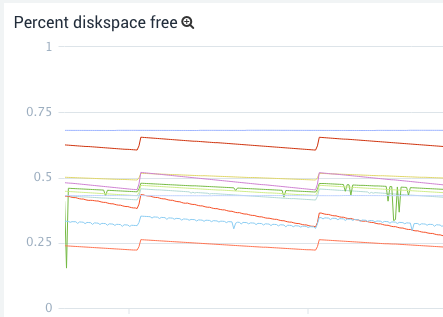Viewing metrics is just as easy as viewing logs. The metric search interface contains a graph for displaying results, text field for entering the query, a tab for setting the axes, and a tab for filtering the results. The language for creating metric queries is the same as for writing log queries and differs only in the operators available. A successful query will return one or more time series, and most of the operators are dedicated to combining and transforming them by group (finding the min value or summing by hostname). Another difference from log searches is that once you have entered a query, a new query box will appear allowing you to run another query and add those time series to the graph as well. Queries can be referenced in future queries as well and you may perform mathematical operations to create new time series that are combinations of old ones. For example, this allows you to create a graph displaying total CPU usage by writing three queries: a system CPU usage query; a user CPU usage query; and a third query, which adds the previous two results together along hostnames.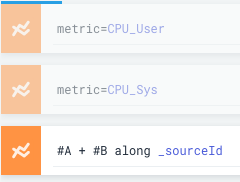The metric search interface also includes a box for entering a log search. When combined with a log search, a bar graph will appear above the time series results to help correlate between trends in the time series and spikes in the number of matching logs.

Metric searches are saved and scheduled a little differently than log searches. Rather than being saved in the library, there is a metric alert screen that lists all previously created alerts, and it is from there that new metric searches can be created and saved (although a search created in the metric search interface can be automatically filled out in the metric alert interface). Metric alerts are a little more powerful than scheduled log searches because they can have multiple connections enabled at a time for their results (email, webhook, etc.), and have both critical and warning conditions.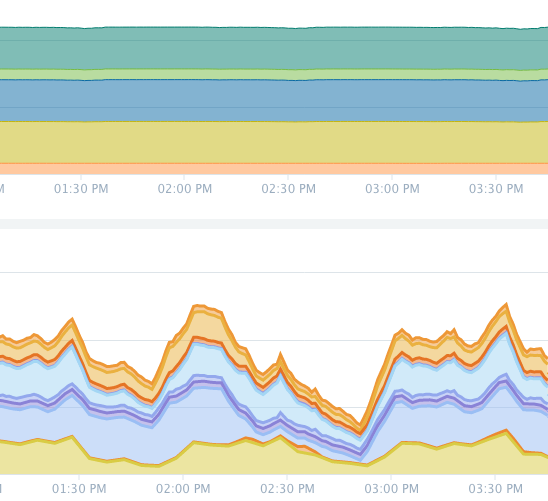Besides saving individual log searches for review later, searches of both types can be saved to panels in dashboards so that less experienced users can get a quick view of what’s going on across your systems without having to write their own searches. Dashboards normally operate in a mode where they must be refreshed manually to view new results, but they can also be operated in live mode where the panels are refreshed as results come in.

Currently, we use Sumo Logic to collect data originating from our applications and infrastructure. This data mostly takes the form of syslog logs, and metrics collected through installed Sumo Logic collectors.

The syslog data is collected in a central place in our infrastructure by a Sumo Logic collector running a syslog server. This is a hold-over from our old system and comes with a slight disadvantage; searching logs is slightly more complicated because as far as Sumo Logic is concerned, the logs come from only one source. This means that messages must contain identifying information, and searches must be written to parse this information. This is not much of an issue in practice because parsing is likely something a log search would contain anyway, but it adds an extra step to learn and increases the complexity of writing a search for a beginner. We have a battery of scheduled searches monitoring the data from our applications which alert based on the source and syslog severity level of the message as well as the presence of some keywords within the message body.

Metrics on the other hand, are ingested by collectors installed on each machine. This means that the metrics can be divided into appropriate categories and Sumo Logic collects metadata from every machine that makes searching easier. The installed collectors can be managed from Sumo Logic’s web interface, making it easy to change which metrics are collected and what category the collectors are in on a per-collector level. The rate at which metrics are collected is also controlled from the web interface, allowing you to control volumes if you are encroaching on your account limits. We had initially tried a solution where all metrics were being funnelled to a single collector running a graphite server but we found the convenience of managing each metric source from the web interface to be very helpful, (more so than for logs) and writing metric searches for this single collector was difficult. Most of our metric alerts monitor the free disk space on our servers and each alert is responsible for monitoring a specific class of device. For example, most of our boot devices have similar sizes and behave similarly so they can all be handled by one alert, while some devices are used for unique services and require their own alerts.

The data we currently collect isn’t of interest on a day-to-day level so currently Sumo Logic’s only role is to monitor and create PagerDuty alerts and Slack messages using web-hooks when something goes wrong. Compared to our old setup, Sumo Logic provides greater flexibility because it is much easier for a user to write their own search to quickly get a time series or log stream of interest, and our dependency on prebaked graphs and streams is diminished. Sumo Logic Dashboards also hold up to our old dashboards and have the advantage of combining graphed metrics with log streams and allowing users to extract a search from a panel so that they can tinker safely in a sandbox.

Our next step is to make better use of Sumo Logic’s analytic resources so that we might provide insight into areas of active research and development. This will require working with teams to identify new sources of data and come up with useful visualizations to help them make decisions relating to the performance of their systems. In expanding the amount of data we are collecting, it might be useful to reduce search time by splitting data up into distinct chunks using Sumo Logic’s partitioning features. Sumo Logic has also announced a logs-to-metrics feature which, when released, could be very valuable to us, as our logs contain numeric data that would be interesting to chart and monitor as if they were metrics.

Sumo Logic may initially appear complicated, but its interface is easy to use and can walk you through writing a query. Sumo Logic is a combined central collection point for logs and metrics, and offers flexibility and greater visibility into our data. If you are interested in using Sumo Logic or would like to know more details about all of its features checkout their website or the docs.

# A Short Note on The Y Combinator

Author: Wessel Bruinsma

This is a cross-post with https://wesselb.github.io/2018/08/16/y-combinator.html.

## Introduction

This post is a short note on the notorious Y combinator. No, not that company, but the computer sciency objects that looks like this:

Don’t worry if that looks complicated; we’ll get down to some examples and the nitty gritty details in just a second. But first, what even is this Y combinator thing? Simply put, the Y combinator is a higher-order function $Y$ that can be used to define recursive functions in languages that don’t support recursion. Cool!

For readers unfamiliar with the above notation, the right-hand side of Equation \eqref{eq:Y-combinator} is a lambda term, which is a valid expression in lambda calculus:

1. $x$, a variable, is a lambda term;
2. if $t$ is a lambda term, then the anonymous function $\lambda\, x : t$ is a lambda term;
3. if $s$ and $t$ are lambda terms, then $s\, t$ is a lambda term, which should be interpreted as $s$ applied with argument $t$; and
4. nothing else is a lambda term.

For example, if we apply $\lambda\, x : y\,x$ to $z$, we find

Although the notation in Equation \eqref{eq:example} suggests multiplication, note that everything is function application, because really that’s all there is in lambda calculus.

Consider the factorial function $\code{fact}$:

In words, if $n$ is zero, return $1$; otherwise, multiply $n$ with $\code{fact}(n-1)$. Equation \eqref{eq:fact-recursive} would be a valid expression if lambda calculus would allow us to use $\code{fact}$ in the definition of $\code{fact}$. Unfortunately, it doesn’t. Tricky. Let’s replace the inner $\code{fact}$ by a variable $f$:

Now, crucially, the Y combinator $Y$ is precisely designed to construct $\code{fact}$ from $\code{fact}'$:

To see this, let’s denote $\code{fact2}=Y\,\code{fact}'$ and verify that $\code{fact2}$ indeed equals $\code{fact}$:

\begin{align} \code{fact2} &= Y\, \code{fact}’ \\ &= (\lambda\, f : (\lambda\, x : f\,(x\, x))\, (\lambda\, x : f\,(x\, x)))\, \code{fact}’ \\ &= (\lambda\, x : \code{fact}’\,(x\, x) )\, (\lambda\, x : \code{fact}’\,(x\, x)) \label{eq:step-1} \\ &= \code{fact}’\, ((\lambda\, x : \code{fact}’\, (x\, x))\,(\lambda\, x : \code{fact}’\, (x\, x))) \label{eq:step-2} \\ &= \code{fact}’\, (Y\, \code{fact}’) \\ &= \code{fact}’\, \code{fact2}, \end{align}

which is exactly what we’re looking for, because the first argument to $\code{fact}'$ should be the actual factorial function, $\code{fact2}$ in this case. Neat!

We hence see that $Y$ can indeed be used to define recursive functions in languages that don’t support recursion. Where does this magic come from, you say? Sit tight, because that’s up next!

## Deriving the Y Combinator

This section introduces a simple trick that can be used to derive Equation \eqref{eq:Y-combinator}. We also show how this trick can be used to derive analogues of the Y combinator that implement mutual recursion in languages that don’t even support simple recursion.

Again, let’s start out by considering a recursive function:

where $g$ is some lambda term that depends on $f$ and $x$. As we discussed before, such a definition is not allowed. However, pulling out $f$,

we do find that $f$ is a fixed point of $h$: $f$ is invariant under applications of $h$. Now—and this is the trick—suppose that $f$ is the result of a function $\hat{f}$ applied to itself: $f=\hat{f}\,\hat{f}$. Then Equation \eqref{eq:fixed-point} becomes

from which we, by inspection, infer that

Therefore,

Pulling out $h$,

where suddenly a wild Y combinator has appeared.

The above derivation shows that $Y$ is a fixed-point combinator. Passed some function $h$, $Y\,h$ gives a fixed point of $h$: $f = Y\,h$ satisfies $f = h\,f$.

Pushing it even further, consider two functions that depend on each other:

\begin{align} f &= \lambda\,x:k_f[x, f, g], & g &= \lambda\,x:k_g[x, f, g] \end{align}

where $k_f$ and $k_g$ are lambda terms that depend on $x$, $f$, and $g$. This is foul play, as we know. We proceed as before and pull out $f$ and $g$:

\begin{align} f = \underbrace{ (\lambda\,f’:\lambda\,g’:\lambda\,x:k_f[x, f’, g’]) }_{h_f} \,\, f\, g = h_f\, f\, g \end{align}

\begin{align}
g = \underbrace{ (\lambda\,f’:\lambda\,g’:\lambda\,x:k_g[x, f’, g’]) }_{h_g} \,\, f\, g = h_g\, f\, g. \end{align}

Now—here’s that trick again—let $f = \hat{f}\,\hat{f}\,\hat{g}$ and $g = \hat{g}\,\hat{f}\,\hat{g}$.1 Then

\begin{align} \hat{f}\,\hat{f}\,\hat{g} &= h_f\,(\hat{f}\,\hat{f}\,\hat{g})\,(\hat{g}\,\hat{f}\,\hat{g}) = (\lambda\,x:\lambda\,y:h_f\,(x\,x\,y)\,(y\,x\,y))\,\,\hat{f}\,\hat{g},\\ \hat{g}\,\hat{f}\,\hat{g} &= h_g\,(\hat{f}\,\hat{f}\,\hat{g})\,(\hat{g}\,\hat{f}\,\hat{g}) = (\lambda\,x:\lambda\,y:h_g\,(x\,x\,y)\,(y\,x\,y))\,\,\hat{f}\,\hat{g}, \end{align}

which suggests that

\begin{align} \hat{f} &= \lambda\,x:\lambda\,y:h_f\,(x\,x\,y)\,(y\,x\,y), \\ \hat{g} &= \lambda\,x:\lambda\,y:h_g\,(x\,x\,y)\,(y\,x\,y). \end{align}

Therefore

\begin{align} f &= \hat{f}\,\hat{f}\,\hat{g} \\ &= (\lambda\,x:\lambda\,y:h_f\,(x\,x\,y)\,(y\,x\,y))\, (\lambda\,x:\lambda\,y:h_f\,(x\,x\,y)\,(y\,x\,y))\, (\lambda\,x:\lambda\,y:h_g\,(x\,x\,y)\,(y\,x\,y)) \\ &= Y_f\, h_f\, h_g \end{align}

where

Similarly,

Dang, laborious, but that worked. And thus we have derived two analogues $Y_f$ and $Y_g$ of the Y combinator that implement mutual recursion in languages that don’t even support simple recursion.

## Implementing the Y Combinator in Python

Well, that’s cool and all, but let’s see whether this Y combinator thing actually works. Consider the following nearly 1-to-1 translation of $Y$ and $\code{fact}'$ to Python:

Y = lambda f: (lambda x: f(x(x)))(lambda x: f(x(x)))
fact = lambda f: lambda n: 1 if n == 0 else n * f(n - 1)


If we try to run this, we run into some weird recursion:

>>> Y(fact)(4)
RecursionError: maximum recursion depth exceeded


Eh? What’s going? Let’s, for closer inspection, once more write down $Y$:

After $f$ is passed to $Y$, $(\lambda\, x : f\,(x\, x))$ is passed to $(\lambda\, x : f\,(x\, x))$; which then evaluates $x\, x$, which passes $(\lambda\, x : f\,(x\, x))$ to $(\lambda\, x : f\,(x\, x))$; which then again evaluates $x\, x$, which again passes $(\lambda\, x : f\,(x\, x))$ to $(\lambda\, x : f\,(x\, x))$; ad infinitum. Written down differently, evaluation of $Y\, f\, x$ yields

which goes on indefinitely. Consequently, $Y\, f$ will not evaluate in finite time, and this is the cause of the RecursionError. But we can fix this, and quite simply so: only allow the recursion—the $x\,x$ bit—to happen when it’s passed an argument; in other words, replace

Subsituting Equation \eqref{eq:strict-evaluation} in Equation \eqref{eq:Y-combinator}, we find

Translating to Python,

Y = lambda f: (lambda x: f(lambda y: x(x)(y)))(lambda x: f(lambda y: x(x)(y)))


And then we try again:

>>> Y(fact)(4)
24

>>> Y(fact)(3)
6

>>> Y(fact)(2)
2

>>> Y(fact)(1)
1


Sweet success!

## Summary

To recapitulate, the Y combinator is a higher-order function that can be used to define recursion—and even mutual recursion—in languages that don’t support recursion. One way of deriving $Y$ is to assume that the recursive function under consideration $f$ is the result of some other function $\hat{f}$ applied to itself: $f = \hat{f}\,\hat{f}$; after some simple manipulation, the result can then be determined by inspection. Although $Y$ can indeed be used to define recursive functions, it cannot be applied literally in a contemporary programming language; recursion errors might then occur. Fortunately, this can be fixed simply by letting the recursion in $Y$ happen when needed—that is, lazily.

1. Do you see why this is the appropriate generalisation of letting $f=\hat{f}\,\hat{f}$

# A Visual Exploration of Coal and Electricity Generation

Author: Chris Davis

In the US, discussions regarding coal tend to be divisive, and are often driven more by politics than data. In this post, we will take an exploratory dive into detailed public data related to electricity generation from coal, and show how this can be used to better understand these systems and the changes they have been undergoing in recent years.

We will look specifically at aspects related to the life cycle of coal, from its origin in coal mines, to its shipments within and between regions, and on to its final use in coal-fired power plants. We will also focus on coal use for electricity generation, as the US electric power sector is responsible for about 90% of overall consumption of coal.

The data we’re going to look at is published by the U.S. Energy Information Administration and is publicly available. In particular, we focus on several of the bulk data files that were released as part of the EIA Open Data API.

The raw data is available in a JSON format, and is very detailed, with information on shipments from specific coal mines to power plants, and also on the operating statistics of power plants.

### Coal Production

Here we use the EIA bulk data on coal which can also be browsed online.

The animation below shows the yearly amount of coal produced, both at the level of individual mines, and also aggregated statistics for total mine production per state. From this, one can see that the mines tend to be locally concentrated based on the various coal basins, and also that the amount of production is by no means evenly distributed.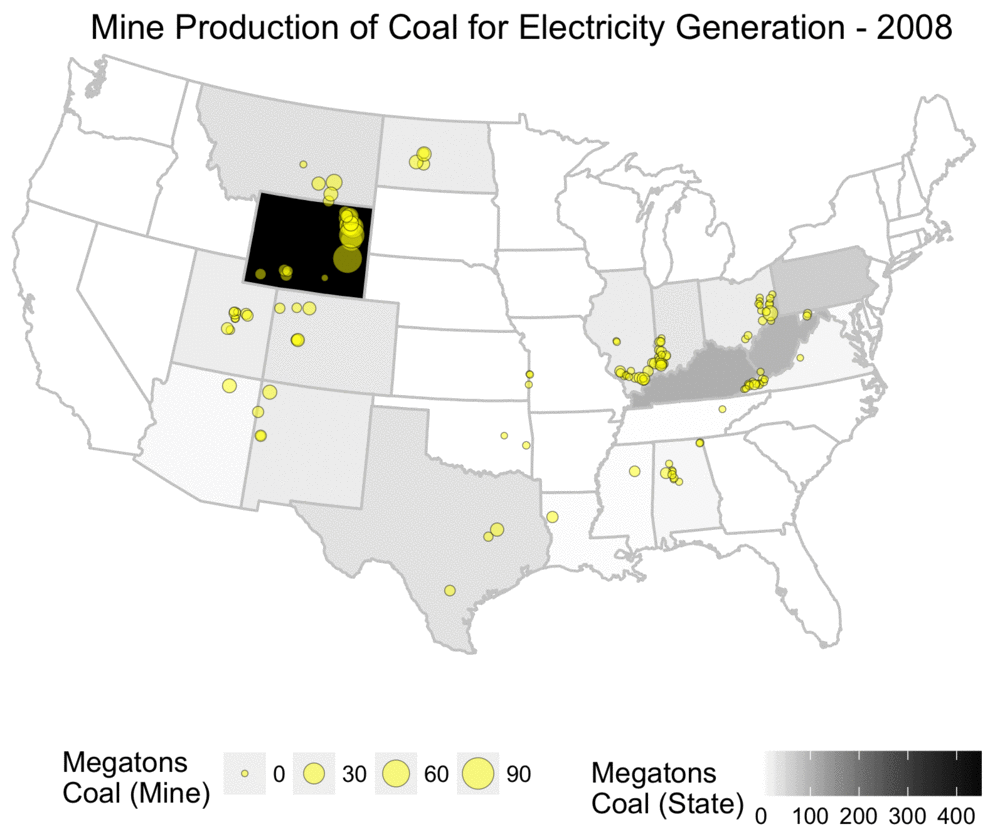Yearly coal production for electricity usage, shown at both mine and state level.

As someone who grew up in the US, this map challenges the common mythology I often heard about Appalachia and coal mining, and assumptions about states such as West Virginia being dominant producers. While Appalachia was a primary source of coal historically, it has been far eclipsed by Wyoming’s Powder River Basin.

The Powder River Basin is composed of surface mines with giant coal seams as shown below. This region produces around 42% of all coal in the US1, with the North Antelope Rochelle Mine alone providing 12% of total US production in 20162. This single mine produces more coal than West Virginia, the second largest coal mining state.Coal seam, North Antelope Rochelle Mine. By Peabody Energy, Inc. CC BY 3.0, via Wikimedia Commons

The animated map below by the Google Earth Engine Timelapse shows the enormous geographic scale of the Power River Basin mines, along with their historical growth over 32 years of satellite imagery. Over time, one can see new areas being dug out with land restoration efforts following shortly behind.

Coal Mines, Powder River Basin, Wyoming, Google Earth Engine Timelapse

#### Sulfur Content

The EIA data on coal shipments has incredible resolution, and one can find information about quarterly shipments between individual mines and power plants. For each of these there is information about the type of coal, ash content, heat content, price, quantity, and sulfur content.

The sulfur content of coal is a concern due to SO2 pollution resulting from coal combustion, which can lead to problems such as acid rain, respiratory problems, and atmospheric haze. While high sulfur content does not necessarily translate into high SO2 emissions due to desulfurization technology used by power plants to reduce emissions3, the process of desulfurization is an economic cost rather than a benefit, and examining sulfur content can at a minimum give indications related to the economics of coal prices.

The plot below gives an overview of how the coal produced each year differs in the amount of sulfur content. To construct the plot, we did the following:

• Per year, sort all coal shipments from those with the highest percentage of sulfur to those with the lowest.
• Using this order, calculate the cumulative quantity. The last value is the total amount of coal produced in the US in that year.

A useful feature of this type of plot is that the area under the curve is the total amount of sulfur contained in coal shipments that year. Instead of reducing the yearly amount of sulfur to a single number, this plot shows how it is distributed based on the properties of the coal shipped.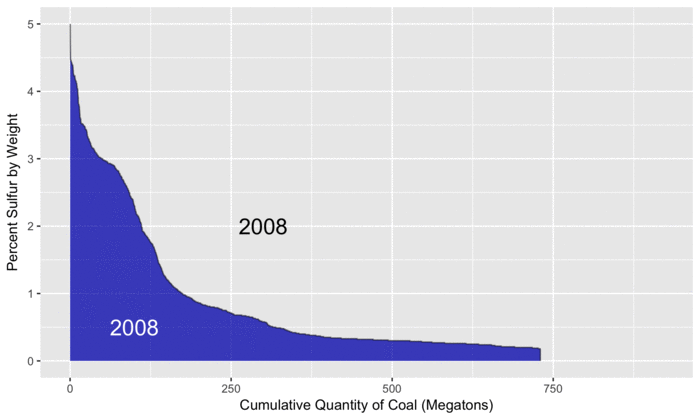Profile of coal sulfur content.

For reference, we use 2008 (in blue) as a baseline since that is the first year in the EIA data. As the animation progresses, we can see that total coal production peaks in 2010, before steadily decreasing to levels below 2008. By examining the differences between the two curves, we can see where increases and decreases in sulfur from different types of coal have occurred.

For example, on the left side of the plot, the gray areas show increased amounts of sulfur from coal that is high in sulfur. Later in the animation, we see light blue areas, representing decreased amounts of sulfur from low-sulfur coal (and less coal production overall). By subtracting the size of the light blue areas from the gray areas, we can calculate the overall change in sulfur, relative to 2008.

As described further below in this post, electricity generation from coal has decreased, although it has been observed that SO2 emissions have fallen quicker than the decrease in generation, in part due to more stringent desulfurization requirements between 2015 and 2016. The increased production of high-sulfur coal shown in the plot suggests an economic tradeoff, which would be interesting to explore with a more detailed analysis. For example, while low-sulfur coal commands a higher price, one could also choose high-sulfur coal, but then be faced with the costs of operating the required desulfurization technologies.

### Transport

After looking at where coal is produced and some of its properties, we will now examine how much is shipped between different regions. To visualize this, we use an animated Chord Diagram, using code adapted from an example showing international migrations.

This technique allows us to visually organize the shipments between diverse regions, with the width of the lines representing the size of the shipments in millions of tons. The axes show the total amount of coal produced and consumed within that year. Arrows looping back to the same region indicate coal produced and consumed in the same region.

To prevent the visualization from being overly cluttered, we group the US states based on US Census Divisions, with the abbreviations for states in each division indicated.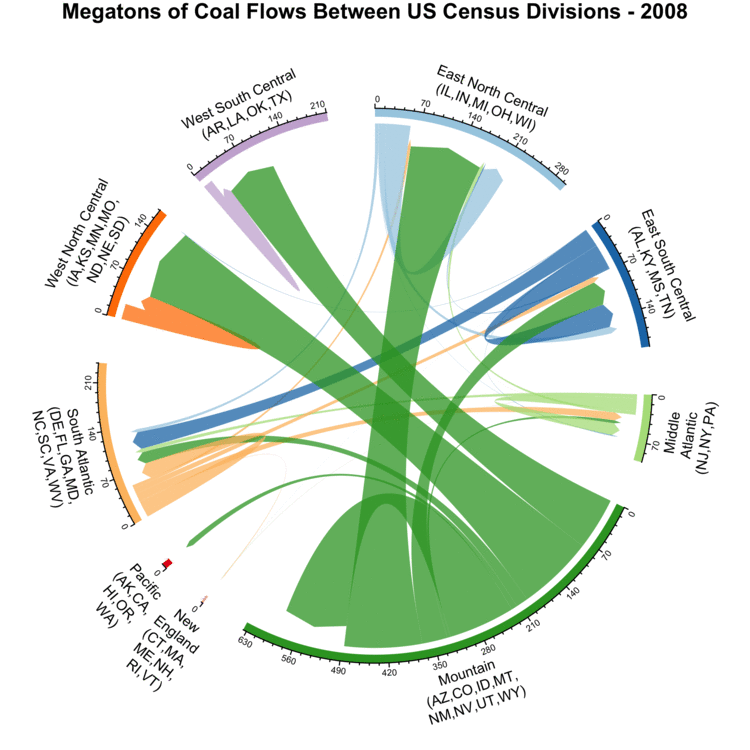Yearly coal flows between different US Census Divisions.

In the three divisions at the top of the plot (West North Central, West South Central, and East North Central), the majority of coal is sourced from states in the Mountain division. The locations of the top five US coal producing states on the plot are indicated below. This list uses statistics from the EIA for 2016, and includes the total amount of production in megatons, along with their percent contribution to overall US production:

• Wyoming: 297.2 MT (41%) - Mountain (bottom)
• West Virginia: 79.8 MT (11%) - South Atlantic (left)
• Pennsylvania: 45.7 MT (6%) - Middle Atlantic (right, below middle)
• Illinois: 43.4 MT (6%) - East North Central (top right)
• Kentucky: 42.9 MT (6%) - East South Central (right, above middle)

Overall, coal shipments have been steadily decreasing since a peak around 2010. Most of the different regions are not self-sufficient, with shipments between regions being common. Only Mountain is self-sufficient, and it also serves as the dominant supplier in other regions as well. Looking a bit deeper, checking the annual coal production statistics for the Powder River Basin reveals that with between 313 and 495 MT of annual shipments, it’s the single area responsible for the vast majority of coal shipments originating from Mountain.

### Coal Consumption

We now look at what happens to the coal once it’s used for electricity generation, and also put this in context of total electricity generation from all fuel sources. For this we use the bulk electricity data, specifically the plant level data which can be browsed online. This data contains monthly information on each power plant, with statistics on the fuel types, amount of electricity generation, and fuel consumed.

While this does not directly document CO2 emissions, we can still estimate them from the available data. We know how much heat is released from burning fossil fuels at the plant on a monthly basis, in millions of BTUs (MMBTU). This information can be multiplied by emissions factors from the US EPA that are estimates of how many kilograms of CO2 are emitted for every MMBTU of combusted fuel. This step tells us how many kilograms of CO2 are emitted on a monthly basis. By dividing this number of the amount of electricity generation, we then get the CO2 emissions intensity in the form of $\frac{kg\ CO_2}{MWh}$.

In the plot below, we use the same approach as that in the sulfur content plot above:

• Yearly generation is sorted from most CO2 intensive (old coal plants) to the least intensive (renewables).
• Using this sorting, the cumulative total generation is calculated.
• The area under the curve represents the total CO2 emissions for that year.

Here 2001 is used as the reference year. Vertical dashed lines are added to indicate total generation for that year, as nuclear and renewables have zero emissions and their generation contributions would not be visible otherwise on the plot. Also, the y axis is clipped at 1500 kg CO2/MWh to reduce the vertical scale shown. The plants with higher values can be older, less efficient power plants, or plants that have been completely shut down and need to consume extra fuel to bring the equipment back up to operating temperatures.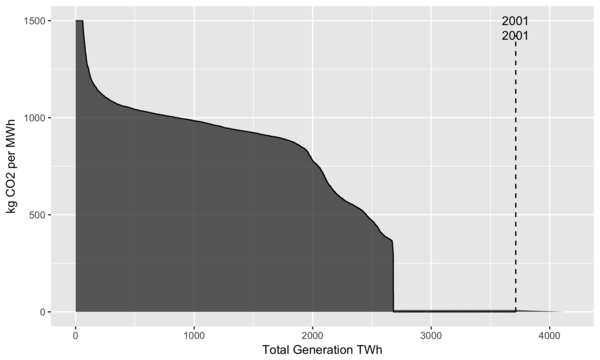Yearly profiles of US electricity generation by carbon intensity.

From the plot we can see that the amount of generation peaked around 2007 and has been roughly stable since then. While some increases in total emissions occurred after 2001, by looking at 2016, we see that generation from fossil fuels is at the same level as it was in 2001. We can also see two horizontal “shelves”, with the lower one around 400 kg CO2/MWh corresponding to generation from natural gas, and the upper one at 900 kg CO2/MWh corresponding to generation from coal4. In 2016, these shelves are quite visible, and the light gray area represents a large amount of emissions that were reduced by switching from coal to natural gas. Overall it’s clear that the US has been steadily decarbonizing the electricity sector.

Another view is shown in the plot below which examines how much of electricity generation is responsible for how much of total CO2 emissions. The motivation for this is that if you find that 90% of CO2 emissions are from 10% of your electricity generation, then large reductions in CO2 emissions can be achieved by changing only a small fraction of the existing infrastructure. This plot uses a similar approach as the previous ones, with the following steps:

• Sort electricity generation per year, from highest CO2 intensity to lowest.
• Calculate total generation and CO2 emissions per year.
• Calculate cumulative generation and CO2 emissions per year.
• Divide these cumulative totals by the yearly totals to get cumulative percentages.

Starting at 2001, this shows that 27% of electricity generation was from nuclear and renewables, with the remaining 73% from fossil fuels. Over time, more renewables (such as large amounts of installed wind capacity), more efficient power plants, and a switch from coal to natural gas have pushed this curve to the left and steepened the slope. As of 2016, 75% of CO2 emissions come from only 35% of electricity generation, with half of CO2 coming from just 21%.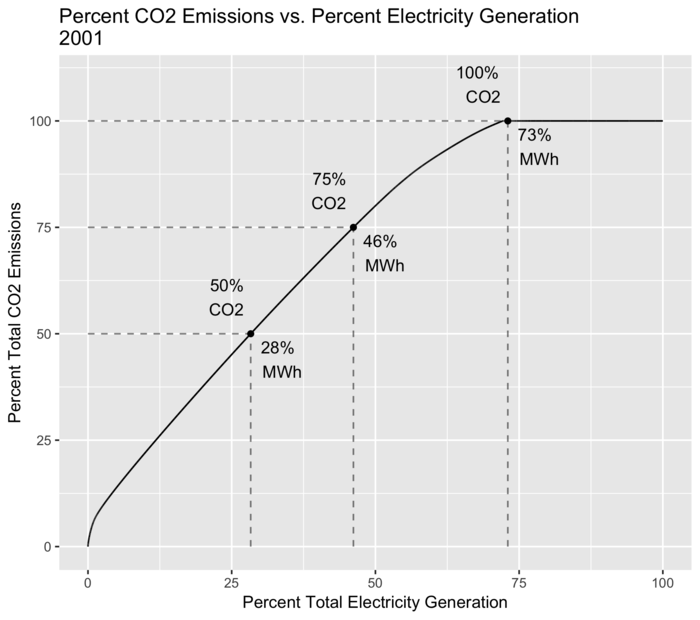Percent of CO2 emissions coming from percent of electricity generation.

### Conclusions

In the above analysis and discussion we looked at only a small subset of what is available in the open data published by the EIA, using a couple of techniques that can help make sense of a deluge of raw data, and tell stories that are not necessarily obvious. Already it is clear that the US is undergoing a large energy transition with a shift towards more natural gas and renewables. As this transition continues to unfold, data such as that published by the EIA will be quite important as we make sense of the resulting environmental and economic impacts.

### Footnotes

1. Calculations based on EIA coal production statistics: Powder River Basin vs. Total US

2. Calculations based on EIA coal production statistics: North Antelope Rochelle Mine vs. Total US

3. This could be more systematically investigated by linking the power plant identifiers in the coal shipments with the US EPA’s Emissions & Generation Resource Integrated Database (eGRID) data, which contains information about actual SO2 emissions from power plants. This would allow us to do a sulfur mass balance to determine how much sulfur arrives from coal, how much sulfur in the form of SO2 leaves into the atmosphere, and how much sulfur is removed in the scrubbing process.

4. Higher values for CO2/MWh can be found in literature, especially if life cycle aspects such as emissions from coal mining, transportation, plant operation, etc. are included. The calculations here are only narrowly focused on the combustion of the fuel and the conversion of heat energy into electrical energy.

# Writing Iterators in Julia 0.7

Author: Eric Davies

With the alpha release of version 0.7, Julia has simplified its iteration interface. This was a huge undertaking which mostly fell to the prolific Keno Fischer, who wrote an entirely new optimizer for the language to accomplish it! As the most active maintainer of the IterTools package, I decided to spend a week rewriting its iterators for the new interface. I’d like to share that experience with you to introduce the new interface and assist in transitioning to Julia 0.7.

## Iteration in Julia 0.6

Previously, Julia’s iteration interface consisted of three methods: start, next, and done. A good way to demonstrate how these work together is to show the transformation from a for loop to the equivalent while loop using those functions. I’ve taken this from the Julia Interfaces documentation, written by Matt Bauman and others.

A simple for loop like this:

for element in iterable
# body
end


was equivalent to this while loop:

state = start(iterable)
while !done(iterable, state)
(element, state) = next(iterable, state)
# body
end


A simple example is a range iterator which yields every nth element up to some number of elements:

julia> struct EveryNth
n::Int
start::Int
length::Int
end

julia> Base.start(iter::EveryNth) = (iter.start, 0)

julia> function Base.next(iter::EveryNth, state)
element, count = state
return (element, (element + iter.n, count + 1))
end

julia> function Base.done(iter::EveryNth, state)
_, count = state
return count >= iter.length
end

julia> Base.length(iter::EveryNth) = iter.length

julia> Base.eltype(iter::EveryNth) = Int


Then we can iterate:

julia> for element in EveryNth(2, 0, 10)
println(element)
end
0
2
4
6
8
10
12
14
16
18


Which is equivalent to:

julia> let iterable = EveryNth(2, 0, 10), state = start(iterable)
while !done(iterable, state)
(element, state) = next(iterable, state)
println(element)
end
end
0
2
4
6
8
10
12
14
16
18


Notice that our EveryNth struct is immutable and we never mutate the state information.

As an aside, the length and eltype method definitions are not necessary. Instead, we could use the IteratorSize and IteratorEltype traits to say that we don’t implement those functions and Julia’s Base functions will not try to call them when iterating. collect is notable for specializing on both of these traits to provide optimizations for different kinds of iterators.

## Iteration in Julia 0.7

In Julia 0.7, the iteration interface is now just one function: iterate. The while loop above would now be written as:

iter_result = iterate(iterable)
while iter_result !== nothing
(element, state) = iter_result
# body
iter_result = iterate(iterable, state)
end


The iterate function has two methods. The first is called once, to begin iteration (like the old start) and also perform the first iteration step. The second is called repeatedly to iterate, like next in Julia 0.6.

The EveryNth example now looks like this:

julia> struct EveryNth
n::Int
start::Int
length::Int
end

julia> function Base.iterate(iter::EveryNth, state=(iter.start, 0))
element, count = state

if count >= iter.length
return nothing
end

return (element, (element + iter.n, count + 1))
end

julia> Base.length(iter::EveryNth) = iter.length

julia> Base.eltype(iter::EveryNth) = Int


In our iterate function we define a default value for state which is used when iterate is called with one argument. 1

This is already less code than the old interface required, but we can reduce it further using another new feature of Julia 0.7.

function Base.iterate(it::EveryNth, (el, i)=(it.start, 0))
return i >= it.length ? nothing : (el, (el + it.n, i + 1))
end


I personally prefer verbosity when it increases readability, but some people prefer shorter code, and that’s easier than ever to achieve.

### A Note on Compatibility

To assist with transitioning between versions, Julia 0.7 includes fallback definitions of iterate which call start, next, and done. If you want code to work on both 0.6 and 0.7, I recommend keeping your iterators defined in those terms, as there isn’t a good way to use the iterate interface on Julia 0.6. Julia 1.0 will remove those fallback definitions and all usage of the old iteration interface.

## Common Strategies

The above example was constructed to be as straightforward as possible, but not all iteration is that easy to express. Luckily, the new interface was designed to assist with situations which were previously difficult or inefficient, and in some cases (like the EveryNth example) reduces the amount of code necessary. While updating IterTools.jl, I came across a few patterns which repeatedly proved useful.

### Wrapping Another Iterable

In many cases, the iterable we want to create is a transformation applied to a caller-supplied iterable. Many of the useful patterns apply specifically to this situation.

#### Early Return

When wrapping an iterable, we usually want to terminate when the wrapped iterable terminates, i.e., return nothing when the wrapped call to iterate returns nothing. If the call to iterate doesn’t return nothing, we want to apply some operations before returning. This pattern was common and simple enough to justify a macro which in IterTools I’ve called @ifsomething2:

macro ifsomething(ex)
quote
result = \$(esc(ex))
result === nothing && return nothing
result
end
end


Putting this code in a multiline macro allows us to simplify code which would usually require multiple lines. This code:

iter = iterate(wrapped, wrapped_state)

if iter === nothing
return nothing
end

val, wrapped_state = iter

# continue processing


becomes this:

val, wrapped_state = @ifsomething iterate(wrapped, wrapped_state)


Conveniently (since it would otherwise error), the value returned from iterate will only be unpacked if it’s not nothing.

#### Slurping and Splatting

The iteration interface requires two methods of iterate, but it’s handy to use default arguments1 to only write out one function. However, sometimes there is no clear initial value for state, e.g., if it requires you to start iterating over the wrapped iterable. In this case it’s helpful to use “slurping” and “splatting”3 to refer to either zero or one function argument—the presence or absence of the state argument.

A simple example is the TakeNth iterator from IterTools.jl. Its implementation of the iterate function looks like this:

function iterate(it::TakeNth, state...)
xs_iter = nothing

for i = 1:it.interval
xs_iter = @ifsomething iterate(it.xs, state...)
state = Base.tail(xs_iter)
end

return xs_iter
end


When you first call iterate(::TakeNth), state starts out as an empty tuple. Splatting this empty tuple into iterate produces the call iterate(it.xs). In all further calls, the actual state returned from iterating over the wrapped iterable will be wrapped in a 1-tuple, and unwrapped in each call.

One of the other tools we use here is the unexported function Base.tail(::Tuple). This function performs the equivalent of slurping on tuples, or xs_iter[2:end]. No matter the size of the input tuple, it will always return at least an empty tuple. This is especially useful in the next, slightly more complicated example.

For TakeNth, we were only passing around the wrapped iterable’s state, but sometimes we need to carry some state of our own as well. For the TakeStrict iterator from IterTools.jl we want to iterate over exactly n elements from the wrapped iterable, so we need to carry a counter as well.

function iterate(it::TakeStrict, state=(it.n,))
n, xs_state = first(state), Base.tail(state)
n <= 0 && return nothing
xs_iter = iterate(it.xs, xs_state...)

if xs_iter === nothing
throw(ArgumentError("In takestrict(xs, n), xs had fewer than n items to take."))
end

v, xs_state = xs_iter
return v, (n - 1, xs_state)
end


Here we use Base.tail to slurp the rest of the input after our counter, so xs_state is either an empty tuple (on the initial iterate call) or a 1-tuple containing the state for the wrapped iterable.

Occasionally we may want to write an iterable that requires advancing the wrapped iterable before returning a value, such as some kind of generic Fibonnaci iterator, or the simplest example, a “peekable” iterator that can look ahead to the next value. This exists in IterTools.jl as PeekIter.

function iterate(pit::PeekIter, state=iterate(pit.it))
val, it_state = @ifsomething state
return (val, iterate(pit.it, it_state))
end


In this case, the work needed for the initial iterate call is just a superset of the regular iterate call, so it’s very simple to implement. In general, the code for look-ahead iterators is just as easy to write in Julia 0.7, but usually more compact.

### Piecewise Development Approach

Having to write many new iterate methods led me to discover some helpful strategies for writing iterate methods when unsure of the best approach. The most helpful thing I did was to write the two-argument method for iterate first, then write the one-argument method, then try to simplify them into a single method. Remember that the one-argument method is a combination of the start and next methods from the old iteration interface. I also realized that it was sometimes easier to apply patterns like the ones above in order to translate from the old to the new iteration interface without attempting to understand the initial version completely.

Let’s look at one of the more complicated iterators in IterTools.jl: Partition. Something that immediately jumps out about the original is this pattern:

if done(it.xs, s)
break
end
(x, s) = next(it.xs, s)


If there are more items, this advances the wrapped iterable, otherwise it breaks out of the surrounding loop. In the new interface this requires just one call instead of two:

iter = iterate(it.xs, s)
iter === nothing && break
(x, s) = iter


Then this pattern can be applied by rote wherever it appears. Applying this and writing two iterate methods results in this4:

function iterate(it::Partition{I, N}, state) where {I, N}
(xs_state, result) = state
# this @ifsomething call handles the 0.6 situation
# where done is always called before next
result[end], xs_state = @ifsomething iterate(it.xs, xs_state)

p = similar(result)
overlap = max(0, N - it.step)
p[1:overlap] .= result[it.step .+ (1:overlap)]

# when step > n, skip over some elements
for i in 1:max(0, it.step - N)
xs_iter = iterate(it.xs, xs_state)
xs_iter === nothing && break
_, xs_state = xs_iter
end

for i in (overlap + 1):(N - 1)
xs_iter = iterate(it.xs, xs_state)
xs_iter === nothing && break

p[i], xs_state = xs_iter
end

return (tuple(result...), (xs_state, p))
end

function iterate(it::Partition{I, N}) where {I, N}
result = Vector{eltype(I)}(undef, N)

result, xs_state = @ifsomething iterate(it.xs)

for i in 2:(N - 1)
result[i], xs_state = @ifsomething iterate(it.xs, xs_state)
end

return iterate(it, (xs_state, result))
end


This works for almost every case, except when N == 1! In that case, we do need to start with iterate(it.xs), but we have to return the first item before calling iterate again, so we have to skip the first iteration in the two-argument method. It would be nice to have the methods be this simple chain, but it looks like we need to combine them.

Previously, we’ve been able to come up with a sensible default state (or a tuple we can splat) for the combined method. We can’t5 do that here, as we need to have conditional behaviour for both cases. Luckily, we can add nothing as a sentinel and Julia will compile the check away. Making this change results in the version which appears in IterTool 1.0:

function iterate(it::Partition{I, N}, state=nothing) where {I, N}
if state === nothing
result = Vector{eltype(I)}(undef, N)

result, xs_state = @ifsomething iterate(it.xs)

for i in 2:N
result[i], xs_state = @ifsomething iterate(it.xs, xs_state)
end
else
(xs_state, result) = state
result[end], xs_state = @ifsomething iterate(it.xs, xs_state)
end

p = similar(result)
overlap = max(0, N - it.step)
p[1:overlap] .= result[it.step .+ (1:overlap)]

# when step > n, skip over some elements
for i in 1:max(0, it.step - N)
xs_iter = iterate(it.xs, xs_state)
xs_iter === nothing && break
_, xs_state = xs_iter
end

for i in (overlap + 1):(N - 1)
xs_iter = iterate(it.xs, xs_state)
xs_iter === nothing && break

p[i], xs_state = xs_iter
end

return (tuple(result...)::eltype(Partition{I, N}), (xs_state, p))
end


## Conclusion

These are the techniques that helped me in my work, but I’d like to hear about more! I’m also curious which patterns improve or harm performance and why. IterTools will definitely accept pull requests, and I’m interested in feedback on Slack and Discourse.

1. In Julia, this actually defines two methods of iterate, as described in the Julia docs 2

2. This name is definitely up for debate!

3. Slurping refers to how using args... in a function definition “slurps” up the trailing arguments, and splatting is the inverse operation. The Julia docs say more on this.

4. All other changes here are renaming or respelling something that appears in the original, for clarity’s sake.

5. We could, but we’d need to do something different depending on the length of the tuple, which would add another conditional check in addition to the splatting.

# SyntheticGrids.jl: Part 2

Author: Eric Perim

## Usage

For the package repository, visit Github.

In the first part, we discussed the motivation and model behind SyntheticGrids.jl. In this post we show how to use it.

To use SyntheticGrids.jl, Julia 0.6.1 or newer is required. Once Julia is properly installed, the package can be installed via

julia> Pkg.add("SyntheticGrids")


This should take care of all dependencies. In order to check if the package has been properly installed, use

julia> Pkg.test("SyntheticGrids")


### A (very) simple test example

As an introduction to the package, we start by automatically generating a small, but complete grid.

julia> using SyntheticGrids

julia> grid = Grid(false);


This command generates a complete grid corresponding to the region contained in the box defined by latitude [33, 35] and longitude [-95, -93] (default values). It automatically places loads and generators and builds the transmission line network (we will soon see how to do each of these steps manually). Here, false determines that substations will not be created. Note the addition of the semicolon, ;, at the end of the command. This has just cosmetic effect in suppressing the printing of the resulting object in the REPL. Even a small grid object corresponds to a reasonably large amount of data.

A Grid object has several attributes that can be inspected. First, let’s look at the buses:

julia> length(buses(grid))
137

julia> buses(grid)
id=1,
coords=LatLon(lat=33.71503°, lon=-93.166445°),
voltage=200,
population=87,
connected_to=Set{Bus}(...)
connections=Set{TransLine}(...)
)

julia> buses(grid)[end]
GenBus(
id=137
coords=LatLon(lat=34.4425°, lon=-93.0262°),
generation=56.0
voltage=Real[115.0],
tech_type=AbstractString["Conventional Hydroelectric"],
connected_to=Set{Bus}(...)
connections=Set{TransLine}(...)
pfactor=0.9
summgen=61.8
wintgen=62.0
gens=SyntheticGrids.Generator[SyntheticGrids.Generator(LatLon(lat=34.4425°, lon=-93.0262°), Real[115.0], "Conventional Hydroelectric", 28.0, 0.9, 15.0, 30.9, 31.0, "1H", "OP"), SyntheticGrids.Generator(LatLon(lat=34.4425°, lon=-93.0262°), Real[115.0], "Conventional Hydroelectric", 28.0, 0.9, 15.0, 30.9, 31.0, "1H", "OP")]
)


We see that our grid has a total of 137 buses (see Figure 2 for a visualisation of the result). The first is a load bus (LoadBus). The values of the attributes connected_to and connections are not explicitly printed. However, the printing of (...) indicates that those sets have been populated (otherwise, they would be printed as ()).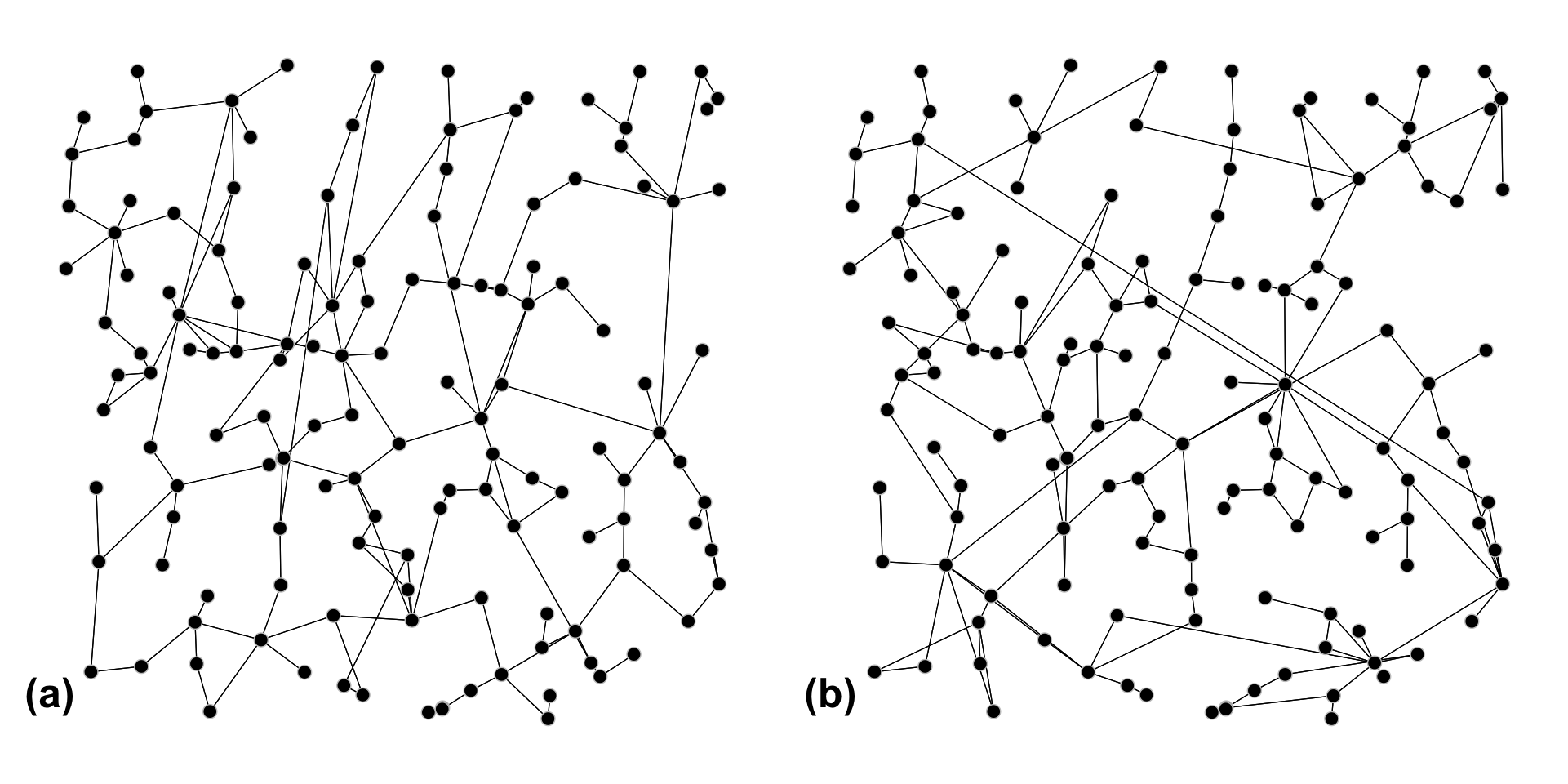Visualisation of two grids generated using the procedure described here. Notice that both present the same bus locations, as their placement is entirely deterministic. The transmission line topology however is different in each case, as it is generated through an stochastic process. Note that the generated grids are non-planar.

The last bus of the list corresponds to a generator (GenBus). One important thing to notice here is that it contains an attribute called gens, which is an array of Generator-type objects. GenBuses represent power plants, which may (or may not, as is the case here) contain several different generating units. These individual generating units are stored within the gens attribute.

We can also inspect the transmission lines:

julia> length(trans_lines(grid))
167

julia> trans_lines(grid)
TransLine(
id=3,
coords=LatLon(lat=33.889332°, lon=-93.097793°),
voltage=100,
population=4090,
connected_to=Set{Bus}(...)
connections=Set{TransLine}(...)
id=1,
coords=LatLon(lat=33.71503°, lon=-93.166445°),
voltage=200,
population=87,
connected_to=Set{Bus}(...)
connections=Set{TransLine}(...)
)),
impedance=0.9175166312451004,
capacity=1400
)


There are 167 transmission lines in our grid. By looking at the first one, we see that they are defined by a tuple of Bus-type objects (here both are LoadBuses), by an impedance value (here taken as Real, since the package has been developed with DC OPF in mind), and a current carrying capacity value.

The adjacency matrix of the system can also be easily accessed:

julia> adjacency(grid)
137×137 SparseMatrixCSC{Bool,Int64} with 334 stored entries:
[3  ,   1]  =  true
[6  ,   1]  =  true
[15 ,   1]  =  true
[34 ,   1]  =  true
[35 ,   1]  =  true
[4  ,   2]  =  true
⋮
[54 , 135]  =  true
[58 , 135]  =  true
[67 , 135]  =  true
[73 , 136]  =  true
[42 , 137]  =  true
[46 , 137]  =  true


Notice that we use a sparse matrix representation for better efficiency.

Substations can also be inspected, but we did not create any, so the result should be empty:

julia> substations(grid)
0-element Array{SyntheticGrids.Substation,1}


That can be remedied by changing the boolean value when creating the grid:

julia> grid = Grid(true);

julia> length(substations(grid))
43

julia> substations(grid)[end]
Substation(
id=43
coords=LatLon(lat=34.412130070351765°, lon=-93.11856562311557°),
voltages=Real[115.0],
generation=199.0,
population=0,
connected_to=Set{Substation}(...)
grouping=SyntheticGrids.Bus[GenBus(
id=137
coords=LatLon(lat=34.4425°, lon=-93.0262°),
generation=56.0
voltage=Real[115.0],
tech_type=AbstractString["Conventional Hydroelectric"],
connected_to=Set{Bus}(...)
connections=Set{TransLine}(...)
pfactor=0.9
summgen=61.8
wintgen=62.0
gens=SyntheticGrids.Generator[SyntheticGrids.Generator(LatLon(lat=34.4425°, lon=-93.0262°), Real[115.0], "Conventional Hydroelectric", 28.0, 0.9, 15.0, 30.9, 31.0, "1H", "OP"), SyntheticGrids.Generator(LatLon(lat=34.4425°, lon=-93.0262°), Real[115.0], "Conventional Hydroelectric", 28.0, 0.9, 15.0, 30.9, 31.0, "1H", "OP")]
), GenBus(
id=135
coords=LatLon(lat=34.570984°, lon=-93.194425°),
generation=75.0
voltage=Real[115.0],
tech_type=AbstractString["Conventional Hydroelectric"],
connected_to=Set{Bus}(...)
connections=Set{TransLine}(...)
pfactor=0.9
summgen=75.0
wintgen=75.0
gens=SyntheticGrids.Generator[SyntheticGrids.Generator(LatLon(lat=34.570984°, lon=-93.194425°), Real[115.0], "Conventional Hydroelectric", 37.5, 0.9, 20.0, 37.5, 37.5, "10M", "OP"), SyntheticGrids.Generator(LatLon(lat=34.570984°, lon=-93.194425°), Real[115.0], "Conventional Hydroelectric", 37.5, 0.9, 20.0, 37.5, 37.5, "10M", "OP")]
), GenBus(
id=136
coords=LatLon(lat=34.211913°, lon=-93.110963°),
generation=68.0
voltage=Real[115.0],
tech_type=AbstractString["Hydroelectric Pumped Storage", "Conventional Hydroelectric"],
connected_to=Set{Bus}(...)
connections=Set{TransLine}(...)
pfactor=0.95
summgen=68.0
wintgen=68.0
gens=SyntheticGrids.Generator[SyntheticGrids.Generator(LatLon(lat=34.211913°, lon=-93.110963°), Real[115.0], "Conventional Hydroelectric", 40.0, 0.95, 15.0, 40.0, 40.0, "1H", "OP"), SyntheticGrids.Generator(LatLon(lat=34.211913°, lon=-93.110963°), Real[115.0], "Hydroelectric Pumped Storage", 28.0, 0.95, 15.0, 28.0, 28.0, "1H", "OP")]
)]
)


By changing the boolean value to true we now create substations (with default values; more into that later) and can inspect them.

### A more complete workflow

Let’s now build a grid step by step. First, we start by generating an empty grid:

julia> using SyntheticGrids

julia> grid = Grid()
SyntheticGrids.Grid(2872812514497267479, SyntheticGrids.Bus[], SyntheticGrids.TransLine[], SyntheticGrids.Substation[], Array{Bool}(0,0), Array{Int64}(0,0))


Notice that one of the attributes has been automatically initialised. That corresponds to the seed which will be used for all stochastic steps. Control over the seed value gives us control over reproducibility. Conversely, that value could have been specified via grid = Grid(seed).

Now let’s place the load buses. We could do this by specifying latitude and longitude limits (e.g.: place_loads_from_zips!(grid; latlim = (30, 35), longlim = (-99, -90))), but let’s look at a more general way of doing this. We can define any function that receives a tuple containing a latitude–longitude pair and returns true if within the desired region and false otherwise:

julia> my_region(x::Tuple{Float64, Float64}, r::Float64) = ((x - 33)^2 + (x + 95)^2 < r^2)
my_region (generic function with 1 method)

julia> f(x) = my_region(x, 5.)
f (generic function with 1 method)

julia> length(buses(grid))
3287


Here, my_region defines a circle (in latitude-longitude space) of radius r around the point (33, -95). Any zip code within that region is added to the grid (to a total of 3287) as a load bus. The same can be done for the generators:

julia> place_gens_from_data!(grid, f)

julia> length(buses(grid))
3729


This command adds all generators within the same region, bringing the total amount of buses to 3729.

We can also manually add extra load or generation buses if we wish:

julia> a_bus = LoadBus((22., -95.), 12., 200, 12345)
id=-1,
coords=LatLon(lat=22.0°, lon=-95.0°),
voltage=200,
population=12345,
connected_to=Set{Bus}()
connections=Set{Transline}()
)

julia> length(buses(grid))
3730


The same works for GenBuses.

Once all buses are in place, it is time to connect them with transmission lines. This can be done via a single function (this step can take some time for larger grids):

julia> connect!(grid)

julia> length(trans_lines(grid))
0


This function goes through the stochastic process of creating the system’s adjacency matrix, but it does not create the actual TransLine objects (hence the zero length). That is done via the create_lines! function. Also note that connect! has several parameters for which we adopted default values. For a description of those, see ? connect.

Before we create the lines, it is interesting to revisit adding new buses. Now that we have created the adjacency matrix for the network, we have two options when adding a new bus: either we redo the connect! step in order to incorporate the new bus in the grid, or we simply extend the adjacency matrix to include the new bus (which won’t have any connections). This is controlled by the reconnect keyword argument that can be passed to add_bus!. In the former case, one uses reconnect = false (the default option); connections can always be manually added by editing the adjacency matrix (and the connected_to fields of the involved buses).

Once the adjacency matrix is ready, TransLine objects are created by invoking the create_lines! function:

julia> SyntheticGrids.create_lines!(grid)

julia> length(trans_lines(grid))
4551


We have generated the connection topology with transmission line objects. Finally, we may want to coarse-grain the grid. This is done via the cluster! function, which receives as arguments the number of each type of cluster: load, both load and generation or pure generation. This step may also take a little while for large grids.

julia> length(substations(grid))
0

julia> cluster!(grid, 1500, 20, 200)

julia> length(substations(grid))
1700


At this point, the whole grid has been generated. If you wish to save it, the functions save and load_grid are available. Please note that the floating-point representation of numbers may lead to infinitesimal changes to the values when saving and reloading a grid. Besides precision issues, they should be equivalent.

julia> save(grid, "./test_grid.json")



Some simple statistics can be computed over the grid, such as the average node degree and the clustering coefficient:

julia> mean_node_deg(adjacency(grid))
2.4402144772117964

0.08598360707539486


The generated grid can easily be exported to pandapower in order to carry out powerflow studies. The option to export to PowerModels.jl should be added soon.

julia> pgrid = to_pandapower(grid)
PyObject This pandapower network includes the following parameter tables: